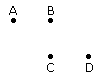Verbal Reasoning - Direction Sense Test - Discussion

Discussion :: Direction Sense Test - Direction Sense 1 (Q.No.28)

28.

Four friends A, B, C and D live in a same locality. The house of B is in the east of A's house but in the north of C's house. The house of C is in the west of D's house. D's house is in which direction of A's house?

 [A]. South-East [B]. North-East [C]. East [D]. Data is inadequate

Explanation:Therefore, D's house is in the South-East direction of A.

 Smita Kharat said: (Mar 25, 2016) Didn't understand this explanation, please explain clearly.

 Priyanshu said: (Jul 6, 2016) Explain it with more details.

 Nivetha said: (Sep 10, 2016) First, we need to understand the pictorial representation. Since B is to the east of A. Mark B to the right of A(east direction). Next, it is given that B is also to the north of C. So mark C below B (north direction). Now we see that AB and C are at right angles. Now since C is to the west of D, mark D on the right side of C such that it is diagonal to B. Now disconnect A with D. We get the required direction.

 Bhagyasri said: (Oct 23, 2016) Here B is in East direction with respect to A. B is in North position with respect to C. C is in West with respect to D. Now we have to consider above-mentioned directional notation. Where is the D's house with respect to A? C is in West and B is in East directions respectively.so A shows north,D shows the south position. We need to give the direction from A to reach D's house. Here A has to go B then only A is reaches to D(D is showing the south-east with respect to A using B s direction)